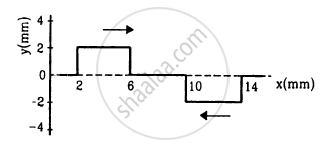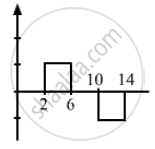Advertisement Remove all ads

# Following Figure Shows Two Wave Pulses at T = 0 Travelling on a String in Opposite Directions with the Same - Physics

Sum

Following figure shows two wave pulses at t = 0 travelling on a string in opposite directions with the same wave speed 50 cm s−1. Sketch the shape of the string at t = 4 ms, 6 ms, 8 ms, and 12 ms.Advertisement Remove all ads

#### SolutionGiven,
Speed of the wave pulse travelling in the opposite direction, v = 50 cm s−1 = 500 mm s−1
Distances travelled by the pulses:
Using s = vt, we get:

$In t = 4 ms = 4 \times {10}^{- 3} s,$

$s = \nu t = 500 \times 4 \times {10}^{- 3} = 2 mm .$

$In t = 6 ms = 6 \times {10}^{- 3} s,$

$s = 500 \times 6 \times {10}^{- 3} = 3 mm .$

$In t = 8 ms = 8 \times {10}^{- 3} s,$

$s = \nu t = 500 \times 8 \times {10}^{- 3} = 4 mm .$

$In t = 12 ms = 12 \times {10}^{- 3} s,$

$s = 500 \times 12 \times {10}^{- 3} = 6 mm .$
The shapes of the string at different times are shown in the above.

Is there an error in this question or solution?
Advertisement Remove all ads

#### APPEARS IN

HC Verma Class 11, 12 Concepts of Physics 1
Chapter 15 Wave Motion and Waves on a String
Q 32 | Page 325
Advertisement Remove all ads
Advertisement Remove all ads
Share
Notifications

View all notifications

Forgot password?